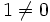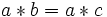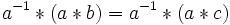# Tour:Inquiry problems two (beginners)

PREVIOUS: Interdisciplinary problems two (beginners)| UP: Introduction two | NEXT: Objective evaluator two (beginners)
PREVIOUS SECTION Inquiry problems: Inquiry problems one|NEXT SECTION Inquiry problems: Inquiry problems three
General instructions for the tour | Pedagogical notes for the tour | Pedagogical notes for this part

## Fixing things that aren't quite groups

### Adding a point at infinity: review

In the previous part, we looked at the following problem:

Consider the set$\R_\infty = \R \cup \{ \infty \}$. Define the following commutative multiplication on$\R_\infty$: the product of two finite real numbers is their usual product, the product of a nonzero real number with$\infty$ is$\infty$ (whichever order we multiply them in), the product of$\infty$ and$\infty$ is$\infty$, and the product of$0$ and$\infty$ is$1$ (whichever order we multiply them in).

Explicitly:

• For$a,b \in \R$, the product$ab$ is defined via the usual multiplication of real numbers.
•$0 \infty = \infty 0 = 1$
•$a \infty = \infty a = \infty \ \forall \ a \in \R_\infty \setminus \{ 0 \}$ ($a$ could be a nonzero real number or$\infty$)
Is$\R_\infty$ a group under multiplication?

In part two, we saw a result that shows this is not possible. We proved that in a group, every element is cancellative. However,$0$ is not cancellative, because$0 * 1 = 0 * 0$, but$1 \ne 0$.

Thus,$\R$ with its usual multiplication cannot be embedded as a subset in any group. In particular,$\R_\infty$ is not a group.

Let's try to see this directly. Why is$\R_\infty$ not a group? There is an identity element, and every element has a two-sided inverse, and multiplication of reals is associative, so where is the problem?

Let's review the proof that in a group, any element is invertible. The proof began like this:$a * b = a * c$.

We then multiply both sides by$a^{-1}$:$a^{-1} * (a * b) = a^{-1} * (a * c)$.

Now, we use associativity:$(a^{-1} * a) * b = (a^{-1} * a) * b$.

And we simplify. The crucial thing we're using is the associativity involving$a$ and$a^{-1}$. This suggests that the problem is with associativity involving$0$ and$\infty$. Indeed:$\infty * (0 * 2) \ne (\infty * 0) * 2$.

### What can be put in a group?

The binary operation of a group is associative and cancellative. Both these properties are inherited to subsets; in particular, any subset of the group closed under the multiplication is a cancellative semigroup.

There are then two reverse questions:

• Given a cancellative semigroup, can it be embedded as a subset of a group, with the same multiplication?
• Given a cancellative monoid, can it be embedded as a subset of a group, with the same multiplication and the same identity element?

Let's try to see some examples where we can do this. Here are two examples:

• Consider the monoid of nonnegative integers under addition. This monoid can be embedded in the group of all integers under addition. Moreover, the group of integers is the smallest group containing this monoid
• Consider the monoid of nonzero integers under multiplication. This monoid can be embedded in the group of all nonzero rational numbers under multiplication. Moreover, the group of rational numbers is the smallest group containing this monoid.
Try to give a general construction that starts with a cancellative monoid, and constructs a group containing it. If you cannot do this, find a construction that works for a particular class of cancellative monoids.

## More about the shape of groups

### Subgroups: small and large

Here's another reason why$\R_\infty$ cannot be a group. If it were, it would have a subgroup,$\R^*$ (the nonzero reals) which misses only two points. This is a very huge subgroup.

Can there exist an infinite group with a subgroup whose set-theoretic complement has size one? Can there exist an infinite group with a subgroup whose set-theoretic complement has size two? Can there exist an infinite group with a proper subgroup whose set-theoretic complement has finite size?

What can we say about the size of a proper subgroup in terms of the size of the whole group? Can we argue that its complement is at least as large as the subgroup itself? Can we say more?